# GANs, a modern perspective

In today’s world, GAN (Generative Adversarial Networks) is an insanely active topic of research and it has already attracted a lot of creative applications like this one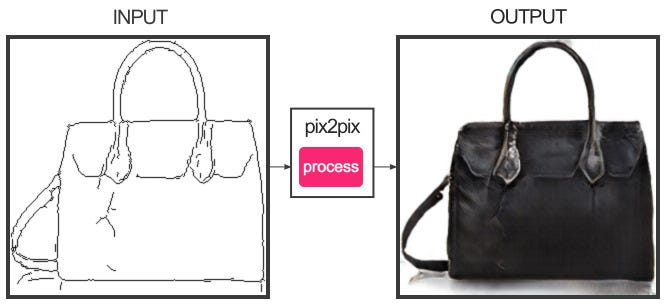Check out pix2pix here

### A brief introduction

#### What’s wrong with today’s deep neural nets ?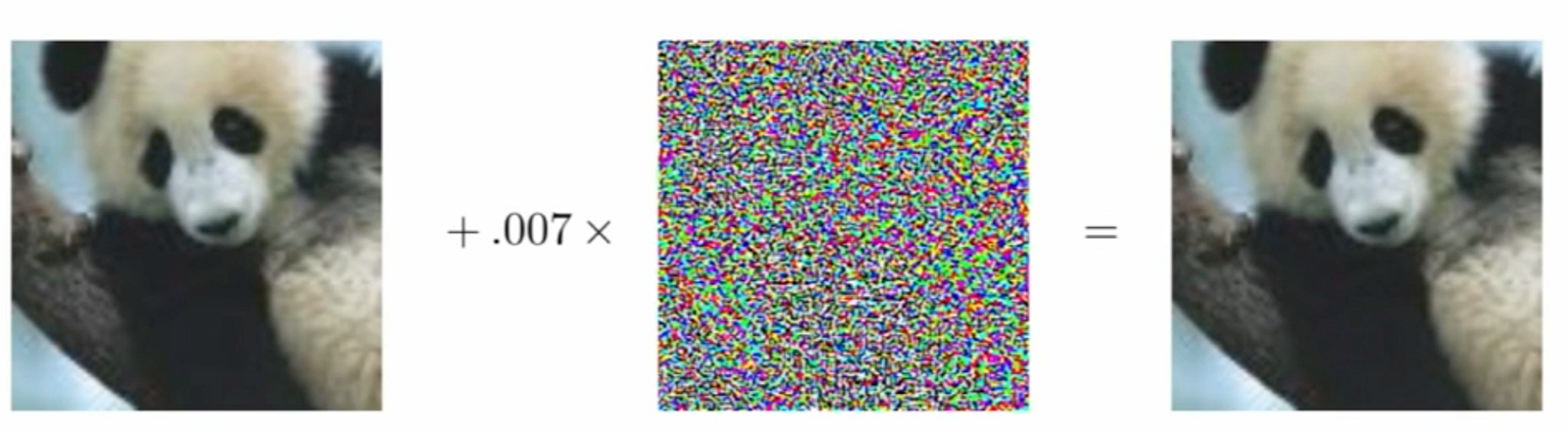Adding some amount of noise to the correctly classified panda image makes a DNN model misclassify it as a gibbon !

It all started in the year 2014 when Christian Szegedy and a couple of others at Google, noticed that neural nets can be fooled easily by adding just a small amount of noise. They used gradient ascent to make their deep neural network classify a given image as something else other than its ground truth class. Surprisingly, they only required a small amount of distortion to convert images of one class to another.

Mathematically, this change of class can be implemented using Fast Gradient Sign Method (FGSM just iteratively adds a small amount noise in the direction of the gradient of the objective function with respect to the input values)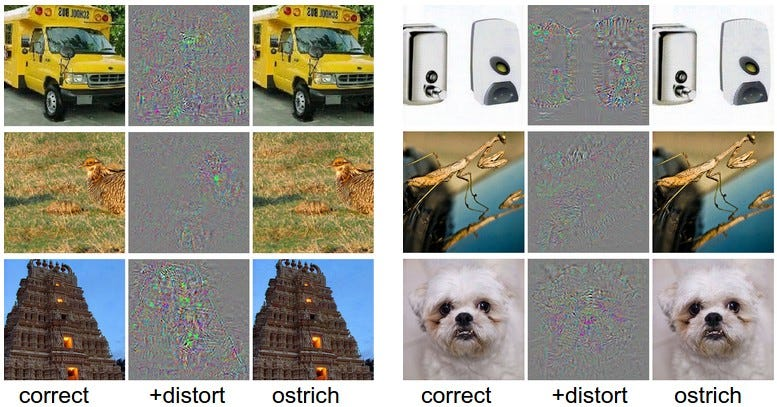The pictures on the left are correctly classified and those on the right (after adding the distort) are misclassified as an ostrich

The most interesting part is that, the model after adding noise has much more confidence in the wrong prediction than that when it predicted right !

#### What are the reasons for such adversaries ?

• Since every machine learning model learns from only a limited number of images for each class, it is prone to overfit.
• Another important reason is that, the mapping between the input to the network and the output is close to being linear. Although we believe that the boundaries of separation between various classes are non-linear, they are composed of linearities and even a small change in a point in the feature space could cause it to cross class boundaries. The activation functions that we use are all mostly piecewise linear too. For example.,ReLu and its variations are all linear after the ‘0’ point.

These are just some of the reasons in layman’s terms. For a clearer understanding about such adversaries, I’d highly recommend this tutorial by Ian Goodfellow.

#### How do we rectify these deep neural nets ?

Well, there’s still a lot of research happening on this and there isn’t a clear cut answer yet. One of the solutions proposed for this was to train the net on adversarial examples as well. And these adversarial examples could be generated using Deep Generative models. There were multiple generative models proposed as well, some of the notable ones being PixelCNN, Variational Auto-encoders and Generative Adversarial Networks (or GANs). In this article, we are particularly gonna explore GANs.

#### What exactly are these “Generative Adversarial Networks” ?

Generative Adversarial Networks consist of a generator and a discriminator neural network. The purpose of the generator is to take in noise vectors and produce images that resembles the input data distribution closely and try to fool the discriminator into classifying a fake image as a real image. The function of the discriminator is to classify a generated image as real or fake. What’s going on between the generator and the discriminator here is a 2 player zero sum game. In other words, in every move, the generator is trying to maximize the chance of the discriminator misclassifying the image and the discriminator is in turn trying to maximize its chances of correctly classifying the incoming image.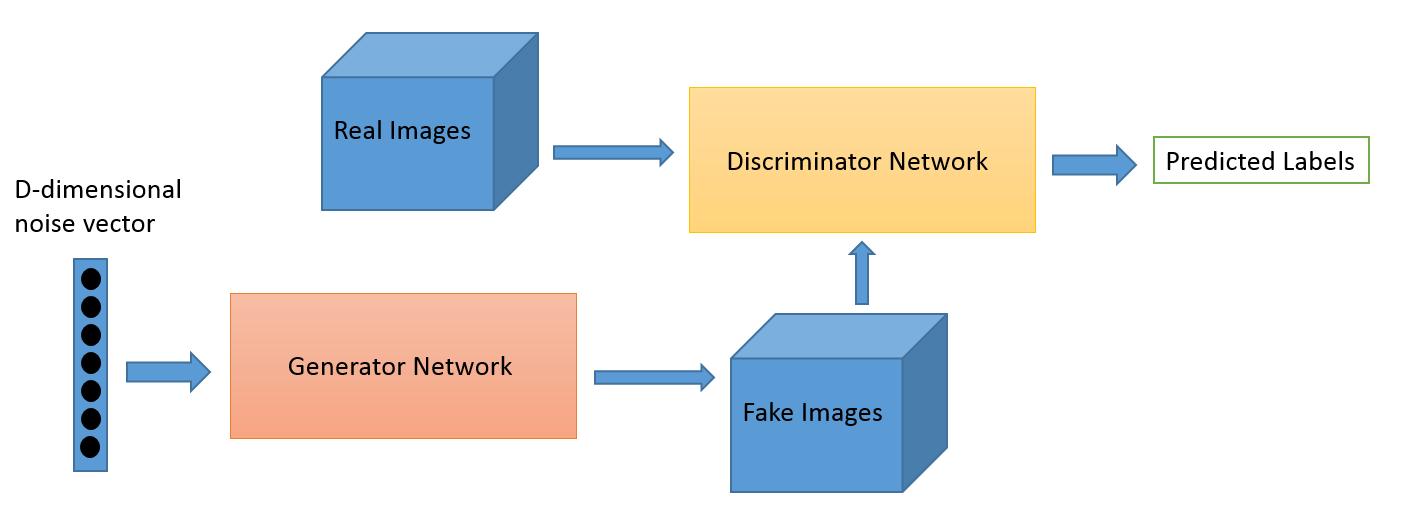A simple flowchart of a GAN

For more information on adversarial examples, do watch this talk by Ian Goodfellow

### Basic Math behind GANs

Obscure isn’t that ? No worries ! it’s easy to understand !

Let’s analyze both the terms in the objective function.

[ Note : θg and θd are just weights of the generator and discriminator networks. You can ignore them while trying to interpret the equations ]

#### Term I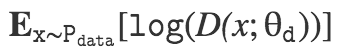Expected Log likelihood of discriminator output when the samples from original data distribution are passed as input

This term represents the Log probability of the discriminator output with input data from real data distribution. Now, look at this term from the Discriminator’s perspective. According to the discriminator, it should maximize its probability of classifying an image correctly as real or fake. Here, the images are sampled from the original data distribution, which is the real data itself. Also, remember that `D(x)` represents the probability that the input image is real. Hence, the discriminator will have to maximize `D(x)` (i.e., it has to be close to ‘1.0’) and `log(D(x))` . And hence, Term I has to be maximized.

#### Term II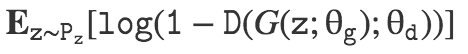Expected Log likelihood of discriminator output when the image(s) from generator’s output are passed onto discriminator

The explanation for this term is quite similar. But you should view this equation from the Generator’s perspective. Here, images from the generator’s output are passed in to the discriminator. So, according to the generator, it has to maximize the chances of the discriminator getting fooled by the generated images. Which means, the generator should want to maximize `D(G(z))` . Which means, it should look to minimize `1 — D(G(z))` and hence `log( 1 — D(G(z))`.

### Types of GANs and their architectures

GANs are one of the hottest research topics today and there are a good number of proposals for GAN implementations in the past couple of years. Here, I’ll discuss only a few of them, although I’ll make sure to list all of the types.

#### Vanilla GAN

This is the simplest type of GAN and in this case the generator and the discriminator are just simple multi-layer perceptrons. Vanilla GANs simply just seek to optimize the mathematical equation using stochastic gradient descent. Let’s take a look at the algorithm.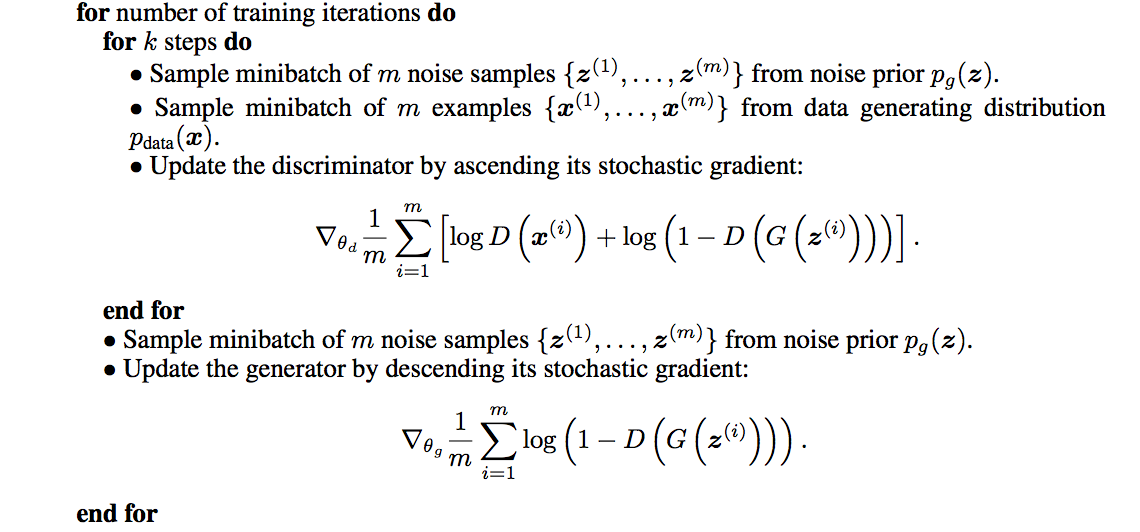This is the algorithm for the very first GAN. It was taken from its paper written in 2014

In layman’s terms, the generator here takes in a noise vector (‘z’, usually 100-dimensional) and produce an image (G(z), which is just a flattened vector of all the pixels in the image). This image is used in the equations we saw previously to simply update the weights of the generator and the discriminator by computing gradients through backpropagation.

#### DCGAN (Deep Convolutional GAN)

This is one of the most popular types of GANs today. In this case, ConvNets are used in place of the multi-layer perceptrons. The objective function remains the same here. Let’s now take a look at the architecture.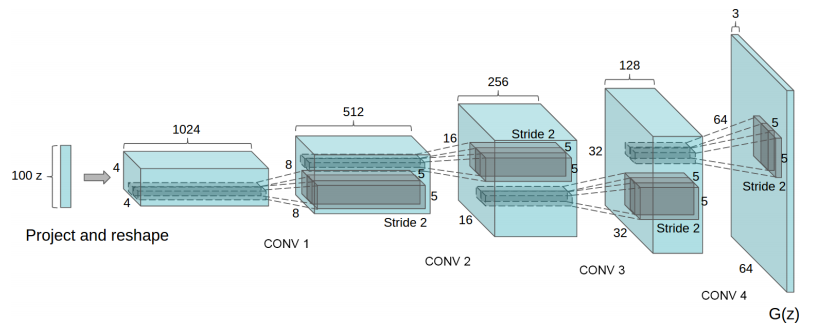Architecture of the generator in the first DCGAN as in its paper

The architecture of the discriminator is mostly just the opposite of that of the generator, i.e., it takes in an image and produces 2 numbers (which are just the probabilities of the image being fake or not). One more thing to note here is that, in the discriminator, the forward process consists of the Conv Transpose or Deconv operation at every layer. Let’s take an example. Let’s say that you wanna map a ‘ 4 X 4 X 1024 ’ layer to a ‘ 8 X 8 X 512 ’ layer and let’s say that we’re using 512 filters of size ‘3 X 3’, then you’ll just have to pad the existing layer’s cross-section with zeroes and add zeroes between each element in the cross-section and do the regular convolution operation with strides. Take a look at the below gif for a clearer understanding.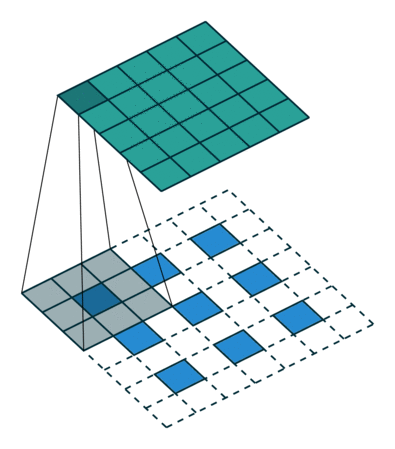A layer with 3 X 3 cross-section is being mapped to a layer with 5 X 5 cross-section

Ok, let’s now take a look at the results that DCGANs have produced.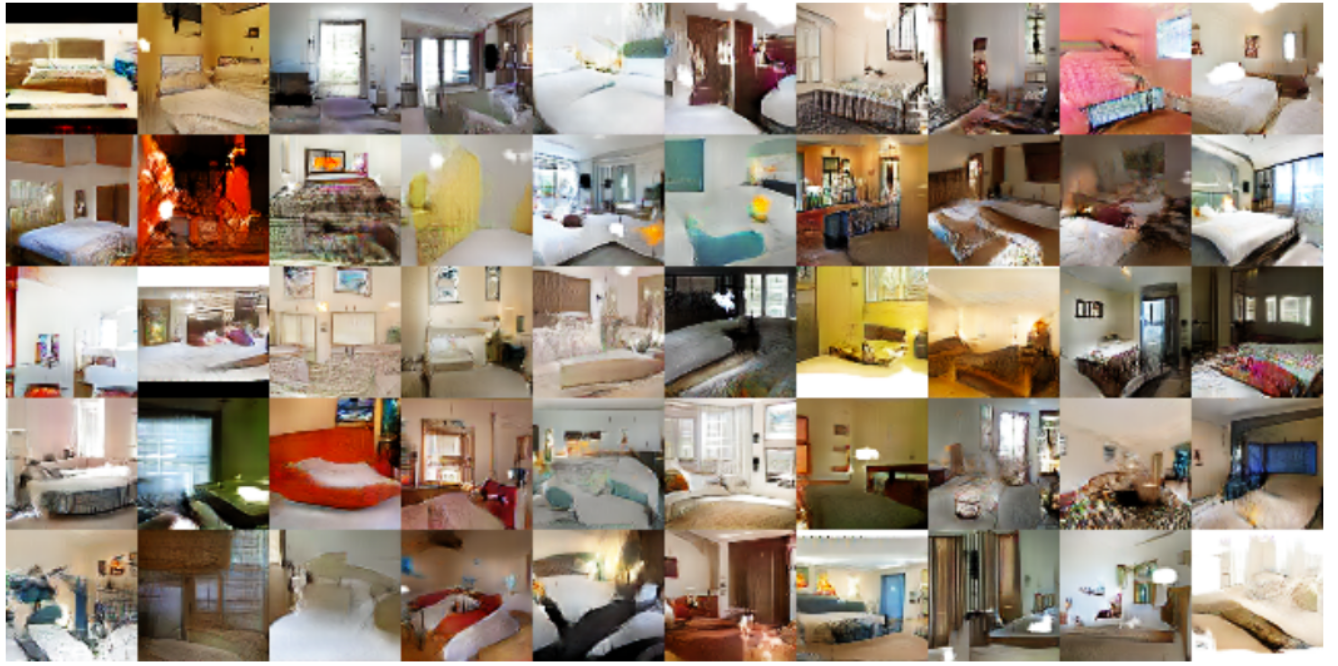This image was taken from the DCGAN paper. This is after 5 epochs of training

#### CGAN (Conditional GAN)

This type of GAN conditions the output data distribution based on a Condition layer. So, in the objective function, `log( 1 — D(G(z))` and `D(x)` will be replaced by `log( 1 — D(G(z|y))` and `D(x|y)` . Rest of it would be the would be taken care of by the respective networks, i.e., creating latent representations and managing weights. You can think of this as more like an additional input of ‘y’ always exists in the networks. And of course, the objective remains same here.

#### LAPGAN (Laplacian Pyramid GAN)

This type of GAN is known to produce very high quality image samples. This uses multiple Generator-Discriminator networks at various levels of a Laplacian Pyramid. Precisely, the image is first downsampled to half its size at each layer of the pyramid. Then, in a backward pass through the pyramid, at each layer, the image acquires a noise generated by a Conditional GAN at that layer and then upsampled to twice its size. This way the image is reconstructed back to its own size.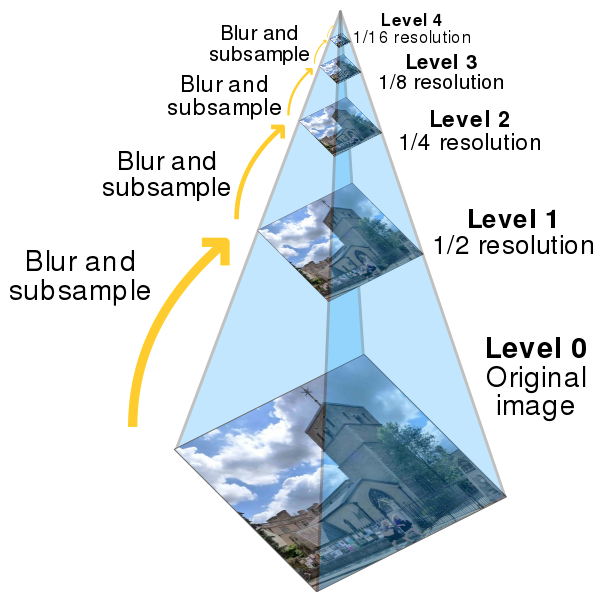Laplacian pyramid

#### Other types of GANs

You can find most types of GANs here :

https://deephunt.in/the-gan-zoo-79597dc8c347

### Problems with GANs and scope for research

• Instability and non-convergence of the objective function in GANs
• Mode collapse (This happens when generator doesn’t produce diverse images ). Here’s a very good article on mode collapse : http://aiden.nibali.org/blog/2017-01-18-mode-collapse-gans/
• The possibility that either the generator or the discriminator becomes too strong as compared to the other during training

### Possible improvements to GANs

• Normalizing the image
• Feature matching : Changing the objective function of generator to MSE w.r.t real data
• Minibatch discrimination : Let the generator classify multiple images in a ‘minibatch’ instead of just one
• Virtual batch normalization : Each example is normalized based on a reference batch of samples

This paper here has a lot of information on improving GANs : https://arxiv.org/pdf/1606.03498.pdf

Phew !